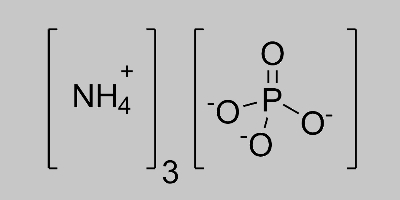# Ammonium Phosphate Formula

Ammonium phosphate formula is a type of a more complex formula that students can come across while studying chemistry. Before learning the formula let us quickly understand what Ammonium phosphate is. It is a type of salt obtained during the reaction between concentrated phosphoric acid and ammonia. The salt is a colourless, odourless, crystalline solid which is not soluble in water. Additionally, it is a highly unstable compound and generally has a less commercial value. However, it is used as a food additive or as an emulsifier, flame retardant and in fertilizers.

## Ammonium Phosphate Chemical Formula

The formula of ammonium phosphate is (NH4)3PO4. The salt compound consists of positively charged ammonium cations (NH4+) and phosphate anion (PO43-) which carries a -3 formal charge. Combining these two ions in the ratio of three to one or cross-multiplying the ionic charges gives us the formula.

 Formula (NH4)3PO4 Molar Mass 149 g/mol Solubility in Water 58.0 g/100 mL

### Ammonium Phosphate Structural Formula

The structural formula of ammonium phosphate can be written as;Stay connected to BYJU’S to learn about the different formulas of important chemical compounds.